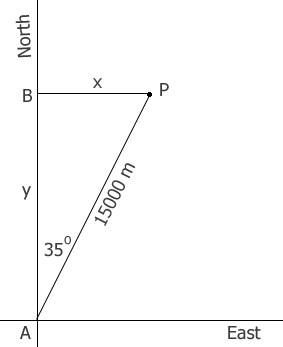SEARCH HOMEMath Central Quandaries & QueriesQuestion from Dennis, a parent: "Air traffic controllers usually describe the position of an aircraft relative to the airport by altitude, horizontal distance, and bearing. Suppose an aircraft is at altitude 500m, distance 15km, and bearing 35 degrees east of north. What are the x,y, and z components (in meters) of the position vector. The x-axis is east, the y-axis is north and the z-axis is vertical.Hi Dennis,

The altitude of the plane is 500 m so the z-component of its position vector is 50 m.

To determine the x and y components I drew a diagram.The airplane is 500 m directly above P, A is the position of the airport, x is the x component of the position vector of the airplane and y is its y component. The horizontal distance AP is 15 km or 15000 m.

What trig function relates the distance from B to A , the distance from A to P and the measure of the angle BAP?

What trig function relates the distance from B toP, the distance from A to P and the measure of the angle BAP?

Solve for x and y.

PennyMath Central is supported by the University of Regina and The Pacific Institute for the Mathematical Sciences.